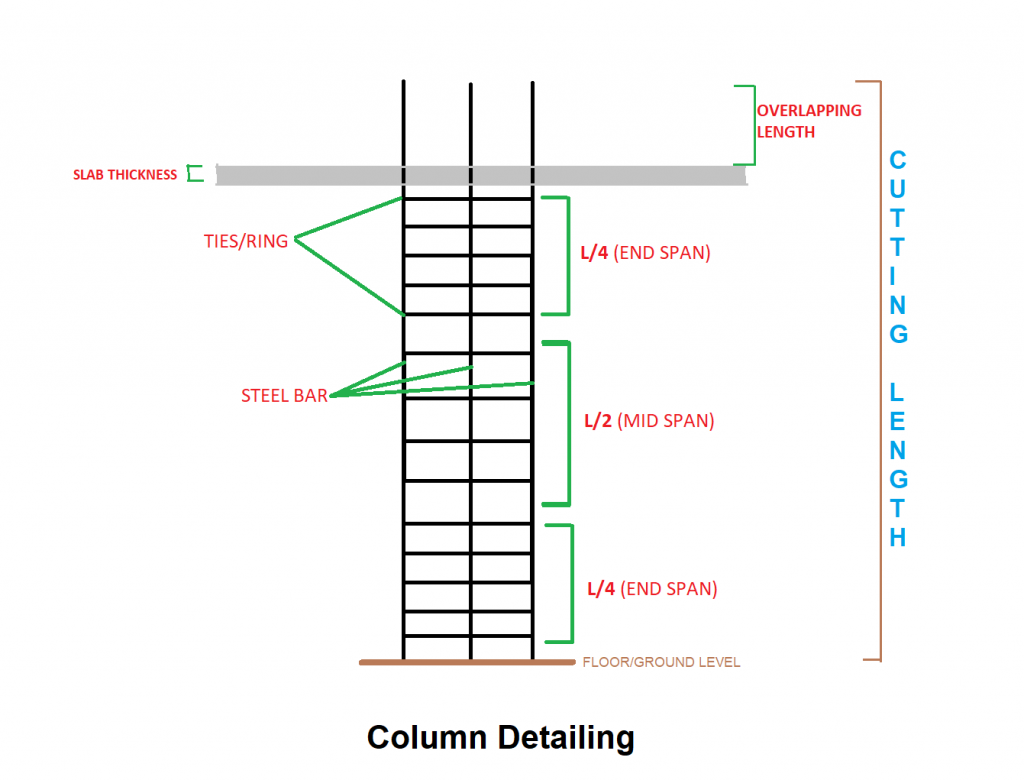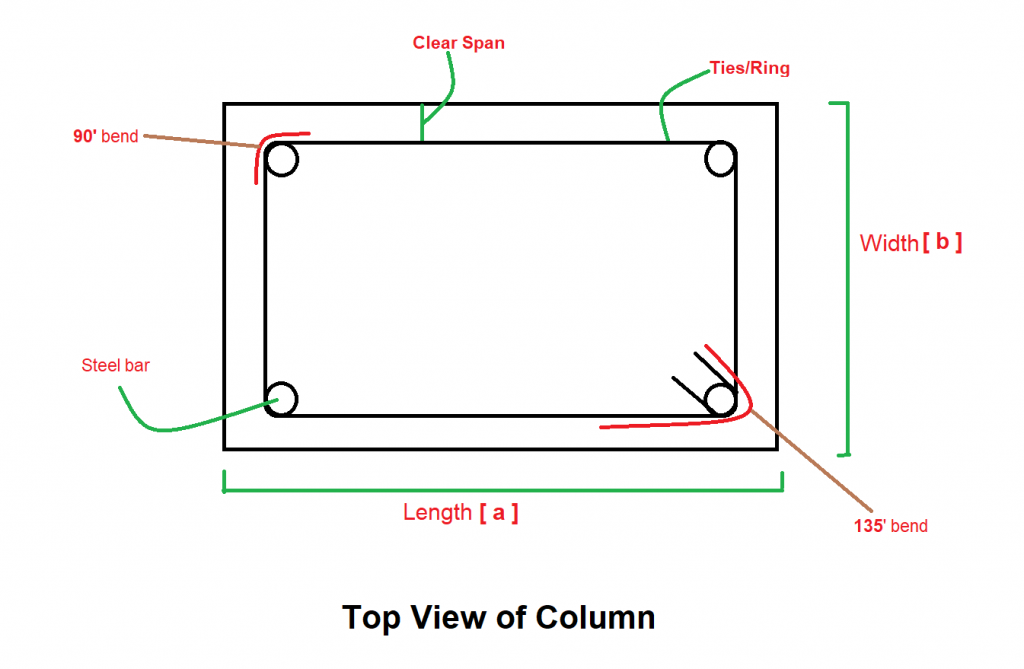Work Hours
Monday to Friday: 7AM - 7PM
Weekend: 10AM - 5PM

# Steel Weight Calculator For Column

## Calculations For Steel Calculator For Column

Let’s understand with the help of an example, how to use the above Steel Calculator for Column.## Part 1: Calculation for Main Bars

Assumptions for Example

• There are 2 types of bars: Bar1 & Bar2
• 4 Nos of Bar1 and 4 Nos of Bar2 are required for the column
• Bar1 Diameter = 20 mm
• Bar2 Diameter = 16 mm
• Height of Slab = Distance between Floor & Slab = 5000 mm
• Slab Thickness = 150 mm
• We are using Steel grade of fe500

Calculation Procedure

• Step 1: Check the Height of Floor on which column to be casted
Length of the Main bar runs parallel with the height of the floor.

• Step 2: Note the Overlap length and Slab thickness (150 mm)
Length of the main bar is an addition of height of the column, height of the top slab and overlap length which is added to the top end of the column for the next floor purpose.
Length of Bar = Height of the Slab + Thickness of the slab + Overlap Length.

• Step 3
Note Diameter of Bars.

• Step 4: Calculate the total length of the main bar
Cutting Length of Bar1 = (5000 + 150 + (50×20)) = 6150 mm
Total Length of Bar1 = 6150 x 4 = 24600 mm
Cutting Length of Bar2 = (5000 + 150 + (50 x 16)) = 5950 mm
Total Length of Bar2 = 5950 x 4 = 23800 mm.

• Step 5: Find the total weight of main bars
Weight of the bar as per their length and diameter by following Formula: –
Unit weight formula for kg per metre =(D^2/163) × L kg/m
Unit weight for feet per metre = (D^2 / 533) × L kg/ft
L is the required length of Steel bar of Diameter D

Result

Weight of Bar1 (4 Nos) = (202/163) x 24.6 = 60.37 KG
Weight of Bar2 (4 Nos) = (162/163) x 23.8 = 37.38 KG

## Part 2: Calculation for Ties & Stirrups

Assumptions For Example

• Length of the column = 450 mm
• Width of the column = 300 mm
• Diameter of Ties= 8mm
• Spacing between Ties at ends = 150 mm
• Spacing between Ties at mid = 200 mm
• Hook Length = 10d or 75 mm
• 90° Bend = 2d
• 135° Bend = 3d
• Concrete Cover = 25 mm

Calculation Procedure

• Step 1: Deduce the Concrete Cover from all sides of tie and find out the length of ties
As shown, [ 2((a-concrete cover) + (b-concrete cover)) ].

• Step 2: Calculate the length (cutting length) of ties including hook and deducting bends (90° & 135°)
Cutting Length of ties = 2((a-concrete cover) + (b-concrete cover)) + 2 numbers of hooks – 3 numbers of 90° bends-2 number of 135° bends
= 2((450-25) + (300-25)) + 2x10x8 -3x2x8 – 2x3x8 = 1464 mm

• Step 3: Then Find out the total no. of ties with the help of Center to the center distance at mid and end span
No. of Ties at mid Span = ((L/2)/ mid-span spacing) + 1
No. of Ties at End Span = 2*[((L/4)/ mid-span spacing) + 1]
No. of Ties = No. of ties at end span + No. of ties at mid span
= 2[((5000/4)/150) +1] + [((5000/2)/200) +1]
=18+14 = 32

• Step 4: Find the total length of ties
Total Length of Ties = 32×1464 = 46848 mm

• Step 5
Note the Diameter of the ties.

• Step 6: Calculate the total weight of ties
Weight of the tie bar as per their length and diameter by following Formula: –
Unit weight formula for kg per metre =(d^2/163) ×L kg/m
Unit weight for feet per metre = (d^2/533)×L kg/ft
L is the required length of Stirrup Steel bar of Diameter d

Result

Total Weight of bar for ties = (82/163) x 49.776 = 18.39 Kg

Final Result

Total Weight of Steel Required = 60.37+37.38+18.39 =116.14 Kg

Try Onsite, #1 Tool For Site Tracking

/** zohoform script */